# Rotational inertia of square about axis perpendicular to its plane

• ChiralSuperfields
I goofed. Should be ##\frac{\sqrt{2}}{2} R##
No worries @erobz! What was the mistake?

Many thanks!

No worries @erobz! What was the mistake?

Many thanks!
##a^2 + a^2 = R^2##

##2a^2 = R^2##

##\implies a = \frac{R}{\sqrt{2}} = \frac{\sqrt{2}}{2} R##

Just carless algebra...late at night for me.

•ChiralSuperfields
##x^2 + x^2 = R^2##

##2x^2 = R^2##

##\implies x = \frac{R}{\sqrt{2}} = \frac{\sqrt{2}}{2} R##

Just carless algebra...late at night for me.
No worries! We all make algebra mistakes! Thank you for your reply @erobz!

So, I'm just trying to understand how you got ## y\hat j = x\hat j##. I have drawn a grey square below.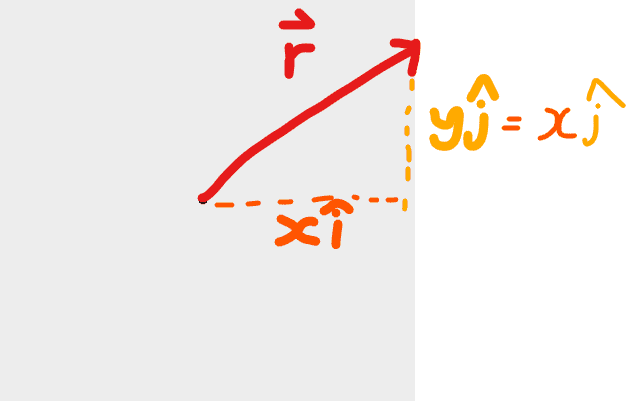I'm trying not to use circular reasoning, but did you get ## y\hat j = x\hat j## because ##\vec r## will always form a right isosceles triangle since it is a square so ##\theta = 45##?

Many thanks!

No worries @erobz! What was the mistake?

Many thanks!
You should be able to work that out yourself. R is the distance from the centre of the square to one corner. How far is it from the centre of the square to the centre of one side? If x is a displacement along a side from its midpoint, what is the range of x?

•ChiralSuperfields
You should be able to work that out yourself. R is the distance from the centre of the square to one corner. How far is it from the centre of the square to the centre of one side? If x is a displacement along a side from its midpoint, what is the range of x?

I was trying to consider cases where ##\vec r ≠ R## for when the position vector is pointing somewhere along to the mass element on the sides not to the corners.

When ##\vec r = R##, I can see that since it square then each side of the right triangle will have equal sides of length ##x##.

I think the range of x for that case would be from ##0## at the midpoint to ##\frac {R}{\sqrt{2}}## at the centre of one side.

Dose this special case hold for ##\vec r ≠ R##?

Many thanks!

I was trying to consider cases where ##\vec r ≠ R## for when the position vector is pointing somewhere along to the mass element on the sides not to the corners.

When ##\vec r = R##, I can see that since it square then each side of the right triangle will have equal sides of length ##x##.

I think the range of x for that case would be from ##0## at the midpoint to ##\frac {R}{\sqrt{2}}## at the centre of one side.

Dose this special case hold for ##\vec r ≠ R##?

Many thanks!
Why do you want to solve this using vectors? It's straightforward using scalars and the parallel axis theorem.

Edit: rereading your post, I don’t understand it. How are you defining ##\vec r## and x?

•ChiralSuperfields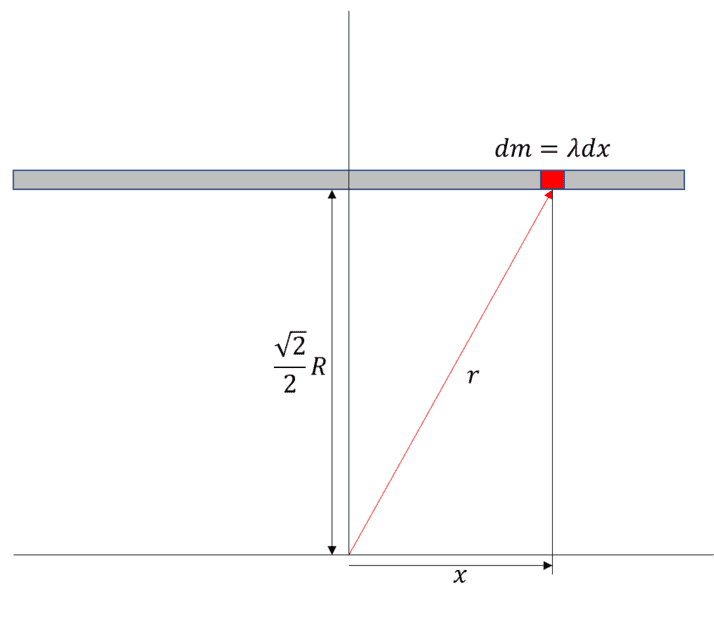•ChiralSuperfields
No worries! We all make algebra mistakes! Thank you for your reply @erobz!

So, I'm just trying to understand how you got ## y\hat j = x\hat j##. I have drawn a grey square below.
View attachment 322331
I'm trying not to use circular reasoning, but did you get ## y\hat j = x\hat j## because ##\vec r## will always form a right isosceles triangle since it is a square so ##\theta = 45##?

Many thanks!
The vector ## \vec{r}## varies, in both magnitude and direction, but for that horizontal segment It will always have the form:

$$\vec{r} = x ~{\hat i} + \frac{\sqrt{2}}{2} R ~{\hat j}$$

•ChiralSuperfields
So you are going to have to change the integrand to what it should be, and your limits of integration.

And to echo @haruspex, do it using @nasu approach (without integration) as a concomitant method.

•ChiralSuperfields
Why do you want to solve this using vectors? It's straightforward using scalars and the parallel axis theorem.

Edit: rereading your post, I don’t understand it. How are you defining ##\vec r## and x?

Sorry, let me try to explain it better.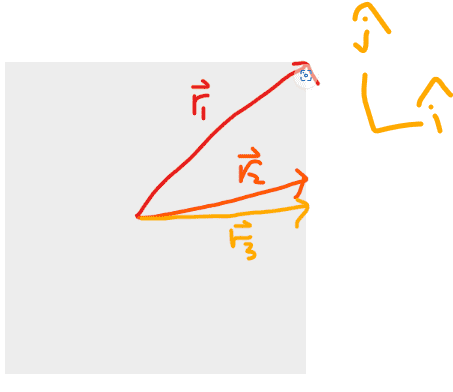(sorry about the tilted coordinate system, the ##\hat i## axis should be horizontal and ##\hat j## axis should be vertical)

##\vec r_1 = \sqrt {x^2 + y_1^2} ## since it has a ##x\hat i## and ##y_1\hat j## component. However since ##y_1 = x## in this special case then ##\vec r_1 = \sqrt {2x^2} = R ##

For this other vector their y-component is not ##x##

##\vec r_2 = \sqrt {x^2 + y_2^2} ≠ R## (cannot equal R since ##\vec r_2 < \vec r_1 ##)

##\vec r_3 = \sqrt {x^2 + y_3^2} ≠ R##

So what I was saying is that we should consider the general case where,

##\vec r = \sqrt {x^2 + y^2} ##

Where x is a constant equal to ##\frac {\sqrt {2}}{2}R## and y is a variable position vector component in the y-direction.

However, if we choose a different coordinate system, such as what @erobz has done in post #43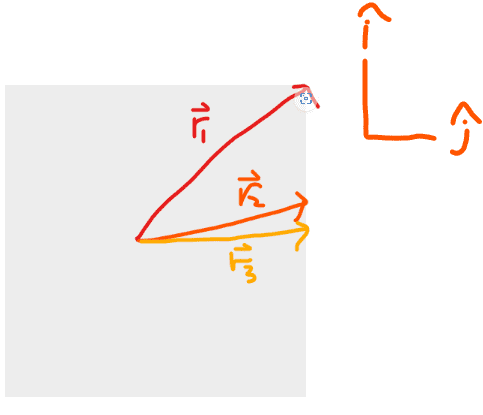Then now y a constant equal to ##\frac {\sqrt {2}}{2}R## and x is variable position vector component in the y-direction.

I think the confusing thing about this problem is choosing the right side to find the moment of inertia for and defining the right coordinate system. What do you think?

How would you solve this using the parallel axis theorem out of curiosity?

Many thanks!

#### Attachments

Last edited:
So you are going to have to change the integrand to what it should be, and your limits of integration.

And to echo @haruspex, do it using @nasu approach (without integration) as a concomitant method.

I am curious to try both approach's :)

Many thanks!

Thank you @erobz!

I think I now understand where you get that constant from now. So basically, you assume that both sides of the triangle are equal which means that angle must be 45 degrees.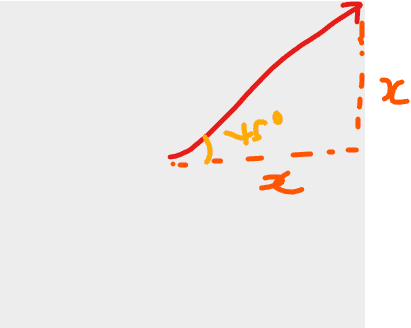So ##\sin45 = x/R## which gives your result.

Is that how you did it?

Many thanks!

The vector ## \vec{r}## varies, in both magnitude and direction, but for that horizontal segment It will always have the form:

$$\vec{r} = x ~{\hat i} + \frac{\sqrt{2}}{2} R ~{\hat j}$$
Thank you for that @erobz!

Thank you @erobz!

I think I now understand where you get that constant from now. So basically, you assume that both sides of the triangle are equal which means that angle must be 45 degrees.
View attachment 322347
So ##\sin45 = x/R## which gives your result.

Is that how you did it?

Many thanks!
Yes, that will always be the case for a square.

•ChiralSuperfields
Won't be easier to just find the moment of inertia for a square of side L and mass m and then use the results for any value of L and for any way this side is related to another parameter in the problem, like to the radius here? You can do this in two or three lines, even by using the integral.
For one side of the square, the moment of inertia relative to the center is just $$\int_{-L/2}^{L/2} [(L/2)^2+x^2]\lambda dx =\lambda L (L/2)^2+\lambda \frac{x^3}{3}\Big|_{-L/2}^{L/2} =\lambda L (L/2)^2+\lambda \frac{2L^3}{3\times 8}$$
I considered the x axis parallel to the side I am looking at.
Using ##M=\lambda L## you get
$$I_{side} =m\frac{L^2}{4}+m \frac{L^2}{12}$$
The last term is the moment of inertia for an axis going through the center of the bar (look it up in a table) and the first term is what you add by using the parallel axis theorem. You could write this right away, without any integral.
Then the monemt of the square is just ##I_{square}=4I_{side}##.
Only now is time to see what happens if ##L=R\sqrt{2}##.

•ChiralSuperfields
Yes, that will always be the case for a square.

Won't be easier to just find the moment of inertia for a square of side L and mass m and then use the results for any value of L and for any way this side is related to another parameter in the problem, like to the radius here? You can do this in two or three lines, even by using the integral.
For one side of the square, the moment of inertia relative to the center is just $$\int_{-L/2}^{L/2} [(L/2)^2+x^2]\lambda dx =\lambda L (L/2)^2+\lambda \frac{x^3}{3}\Big|_{-L/2}^{L/2} =\lambda L (L/2)^2+\lambda \frac{2L^3}{3\times 8}$$
I considered the x axis parallel to the side I am looking at.
Using ##M=\lambda L## you get
$$I_{side} =m\frac{L^2}{4}+m \frac{L^2}{12}$$
The last term is the moment of inertia for an axis going through the center of the bar (look it up in a table) and the first term is what you add by using the parallel axis theorem. You could write this right away, without any integral.
Then the monemt of the square is just ##I_{square}=4I_{side}##.
Only now is time to see what happens if ##L=R\sqrt{2}##.
Thank you very much for that @nasu!

I have done that @nasu!

##I_{side}= \frac {2mR^2}{4} + \frac{2mR^2}{12} ##
##I_{side} = \frac {mR^2}{2} + \frac{mR^2}{6} ##
##I_{square} = 4[\frac {mR^2}{2} + \frac{mR^2}{6}]##
##I_{square} = 2mR^2 + \frac{2mR^2}{3}##
##I_{square} = \frac{8mR^2}{3}##

Many thanks!